# Electronics and Communication Engineering - Electromagnetic Field Theory

16.

The I/P impedance of a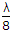long short circuited section of a loss less transmission line is

 A. zero B. inductive C. capacitance D. infinite

Explanation: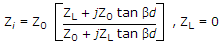if Zi = -jkZ0 then capacitive = jkZ0 the inductive.

17.

Which of the following relations is correct?

 A. ∇ .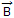= r B. ∇ .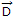= r C. ∇ .= - r D. ∇ .=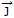Explanation:

No answer description available for this question. Let us discuss.

18.

For frequencies upto 1650 kHz __________ antenna is used

 A. yagi B. grounded C. turnstile D. marconi

Explanation:

No answer description available for this question. Let us discuss.

19.

Due to which of the following reasons, the ground wave eventually disappears, as one moves away from the transmitter?

 A. Tilting B. Loss of line-of-eight conditions C. Interference from the sky wave D. Maximum single hop distance limitation

Explanation:

No answer description available for this question. Let us discuss.

20.

A loop antenna may be of

 A. square on triangular shape B. hexagonal shape C. circular shape D. any of the above